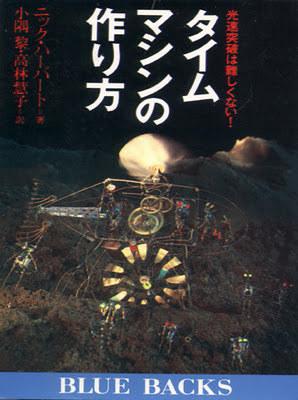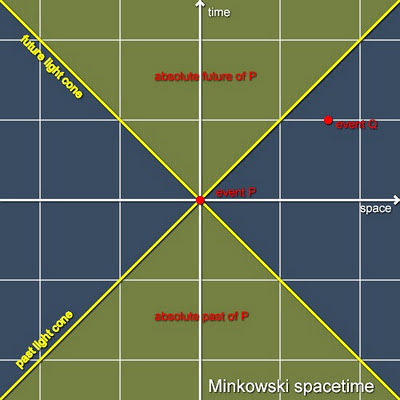## Thursday, November 3, 2011

### Time Machine DesignJapanese Translation of Nick Herbert's Faster Than Light
Starting from the upper right, and reading downward, the characters are phonetic ta-i-mu, but the ending u goes silent, so this is "time" and is written in English (katakana).

The middle column phonetically reads ma-shi-n, or "machine" again written in English.  The bottom character is the phonetic Japanese particle -no- indicating possession.

The top character in the left column is the verb tsuku- which means "to build", and it is followed by a phonetic -ri, turning it into a noun meaning "construction".

The bottom character on the left is kata (the same word as in martial arts), meaning "form".  The composite tsukurikata means "building instructions".

So the whole thing reads time machine no tsukurikata which literally means "time machine's building instructions" -- Cool!

(translation by Alec MacCall, Santa Cruz NOAA scientist and Celtic musician). Thanks, Alec.

=====================================

How could the ability to signal faster-than-light be used to construct a time machine? Here physicist/programmer Richard Baker shows what you would need to send a signal from the present to the past if your FTL signal was infinitely fast--instantaneous communication as in Ursula LeGuin's science-fiction "ansible".

Baker starts by considering the rest frame V = 0 and drawing a Minkowski diagram with time increasing in the upward direction and one spatial dimension plotted in the sidewards direction. In this diagram, the speed-of-light limit divides spacetime into two distinct regions: 1) those events that can be causally connected to point P (which are called "time-like") and 2) those events that are causally disconnected from P--called "space-like". In Baker's diagram time-like events occur in the yellow region, which is called the "light-cone", and space-like events occur in the blue region, called the "absolute elsewhere".

If we could send an instantaneous signal from P, it would travel along the white horizontal axis (t = 0) in FIG 1, but it would not go backwards in time. To build a time machine we need to introduce a second reference frame moving to the right at velocity V with respect to the rest frame.FIG 1 -- Spacetime diagram: Rest Frame V = 0
The fundamental premise of Einstein's theory of special relativity is that space and time look different for observers that are in relative motion. A system of equations called the Lorentz Transformations shows how to translate between the spacetime events as they appear to an observer in the rest frame (V = 0) and to an observer in the frame moving at velocity V.FIG 2 -- V= 1/4 c Frame as seen in V = 0 Rest Frame

Assume that our moving frame is traveling at 1/4 of the speed of light. This moving frame is bounded by the same light cones but in the rest frame the moving frames time and space axes seem tilted as
shown by heavy blue lines in FIG 2. Now if the observer in the moving frame sends an instantaneous signal to the right--the direction of his frame's motion--it will always go forward in time (as seen from the rest frame). However, if the moving observer sends an instant signal in the opposite direction, it will seem (in the rest frame) to go backwards in time.

One way to remember the direction of this effect is to imagine running on an escalator. If you run UP the UP escalator, all you do is go faster. But if you run DOWN the UP escalator fast enough you can go backwards in time.

The signal goes back in time but it is traveling into the rest frame's elsewhere. To construct a time machine in the rest frame we have to bounce that signal back. This is accomplished with a second frame located at distance D traveling at velocity minus V. We do the "DOWN the UP escalator trick" in this second frame and the signal returns to the rest frame BEFORE IT WAS SENT.

Richard Baker concisely derives the time machine effect for FTL signals which travel instantly. I will follow his example (and modify his diagrams) for FTL signals of finite velocity Z where Z is greater than c--the velocity of light. I will derive the "time machine equation" for all frame velocities V and all FTL signaling velocities Z, but in FIG 3 will show the results for only one frame speed (V = 1/4 c) and three FTL speeds (Z = 2c, 4c and 8c).FIG 3 -- FTL signals (Z = 2c, 4c, 8c) in the 1/4 c frame--viewed from the Rest Frame

Deriving the time machine equation is a schoolgirl exercise in special relativity. Lorentz transforming into the moving frame, putting in a slanted line representing a signal traveling at velocity Z, then transforming back to the rest frame, it is easy to see that the "time machine factor" F is given by the simple expression:

F = (ZV - 1)/(Z - V)

when Z and V are expressed in multiples of c, F is a dimensionless number proportional to how far back you go in time when sending an FTL signal Z a distance D from a frame traveling at velocity V. Only if F is positive do signals go backwards in time.

The "one-way time-travel equation" is: T = FD

For example if D = 1 light-year and F = 1/2 then T is 1/2 year backwards in time. Since the time machine is not complete unless we bounce the signal back, this number must be doubled.

The "time machine equation" is: T = 2FD

It is easy to see that the time machine factor will only be positive if Z > 1/V. Therefore for a pair of frames moving at V = 1/4 c, the FTL signal must be faster than 4c for the time machine to work. FIG 3 shows three values of Z. For Z = 2c, the signal goes into the future; for Z = 4c, the signal stays at t = 0, traveling neither into the past nor the future. For Z = 8c, the signal travels into the past. Only the last FTL signal is fast enough to build a time machine.

As the speed Z is made faster and faster, the time machine factor F goes to V. Thus the time machine expression T(instant) for instantaneous (ansible-class) signal speeds is:

T(instant) = 2VD

To put these numbers in perspective, we imagine building a time machine that sends an FTL signal from Earth to the Moon and back that arrives at Earth before it was sent. For our moving frames we use rotating disks whose rim velocities are V = 1/4c from which FTL signals can be sent and received at speed Z. To keep the numbers simple, we assume the Earth-Moon distance to be D = 2 light-seconds.Time Machine operating between Earth and Moon

To operate the time machine we send the FTL signal when the disk is moving in the opposite direction to the motion of the FTL signal (using the DOWN the UP elevator effect) and we receive this signal at a detector moving at the same speed and direction as the sender. When V = 1/4c and Z = 8c, the time machine factor F is 0.129. From the time machine equation we see that the signal will/did arrive at Earth about 1/2 second before it was sent. The signal can be resent N times and progressively displaced 1/2 N second backwards in time but no further back than when the machine was first turned on.

For Z = 8c, the one-bounce time travel is 1/2 second. If the machine were operating in ansible mode (Z = infinity), the time machine factor F is equal to V which in this case is 1/4. Hence in ansible mode the one-bounce time travel interval is 4 x 1/4 = one second backwards in time.

Faster-than-light signaling devices have long been a science-fiction staple, from Ursula LeGuin's ansible to Star Trek's subspace radio. A short review of famous fictional FTL devices can be found here.Artist's conception of Ursula LeGuin's Ansible FTL communicator